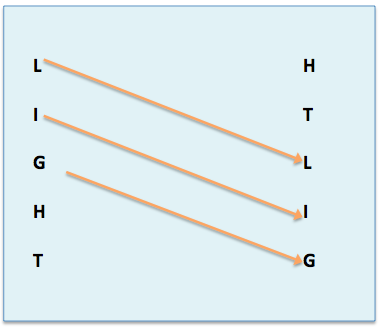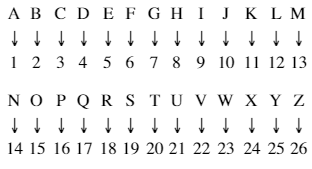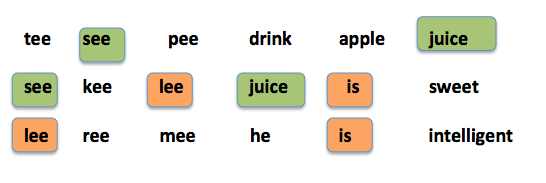# Coding & DecodingCoding & Decoding questions help judge candidate’s ability to understand the logic that codes a particular message and his/her ability to read the message. Question from coding can com from variety of types and so we shall see them one by one.bUt before going into any topic or question, we first need to understand What is significance of Code?
Any Code has essentially two parts: A. Rule of Code B. Message(Public)
Now, anyone can see this public message but the message but none can understand because they do not know rule of code. Why it is important to us students? Well because, you need to understand what question demands and from above we can safely say the questions demand us to “understand the rule of code“.
If you are a person who has to code a secret message, what is the easiest way to do so? the easiest way is to assign alphabets a number.  Number have the ability to add, subtract, divide,  multiple which alphabets can’t. Now, for simple purposes:
Let’s say (picture in right) is the number we assign. Now we have numbers, all we need is rule of code.Let’s say we decide rule of code is “A=1 & +5” a simple term. Now I want to send a secret message, a secret location . I simply draft two letters One with  “A=1 &+5” . Second with  YZGCD and send with 2 separate couriers. Now, each of the letters has no meaning but when the two are put together , one can simply understand:
y+5 = D
Z+5= E
G+5 =L
C+5=H
D+5=I

Now, you must be thinking, all that is good story but what is the use, well, two important take-aways:
1. Primary motive should be finding rule of code.
2. How, Converting alphabets to numbers.
The right picture should form basis of your problem solving and you should try and learn Ranking of each word. Nowadays, as coaching are advanced, RPSC/UPSC/SSC all might also try you with reverse ranking .i.e raking of letters considering z=1.
1. Letter Coding:
They are the most basic types to start with, here the student needs to understand code at Letter Level.
Example: TEACHER is coded as VGCEJGT, here code is working at letter level. i.e. T will have relation to VorG or any other letter. But relation will be letter to letter. It can be of following types:

A.1. Distance Based:
In above, example, we check number codes of Message Letters:
T (20)        V(22)
E(5)            G(7)
A(1)            C(3)
C(3)            E(5)
H(8)           J(10)
E(5)            G(7)
R(18)         T(20)
Now, just by watching, the codes we can understand that rule of Code is A=1 & + 2  . The rank of Letters in 2nd word are +2 the rank of letters of 1st word. or. The distance between T & V (2), G&E(2) is same for all other Letters. Hence these are distance based problems where, once can figure out code by writing ranks of letters next to them.

A.2. END Based:
In such problems, the END positions are used as Coding rule.
Example: TEACHER is coded as GVZXSVI
FROM LEFT (A=1)                        FROM RIGHT(Z=1)
T (20)                                                G(2o)
E(5)                                                    V(7)
A(1)                                                    Z(1)
C(3)                                                   X(3)
H(8)                                                  S(8)
E(5)                                                   V(7)
R(18)                                                 I(18)
Here, the position of letters T  from LEFT(A=1) is same as that of G From Right(Z=1)…and this is the rule of Code.
A.3. Position Swap based
In such problems Position of letters is changed.
LIGHT is coded as HTLIG, now here we should note that no new alphabets are coming  and so we know that it is just rearrangement. Till now, we were stressing on Number & Alphabet relation, in rearrangement  the rule of code is Position based. Hence, decoding position.
And the easiest way for decoding position is making marking arrows:Now you know that each letter is shifted right (2 positions) and this is the rule of code.
A. 4. Number Based:
Returning back to numbers, it is not necessary that Words are coded only to Words and direct Words to number may also be given. Example: Sister is coded as 535201, UNCLE is coded as 78960, then how is TISER coded?
1st Step:
Observation1:Word1: 6 letters = 6 numbers & Word2: 5 letters = 5 numbers, it means each letter has corresponding number to it.
Observation 2: The word asked is made from question words only, hence, relation may not be general (wo+6, type)
2nd Step: Assumption: Straight relation: now given S=5, cannot be not from A=1 or Z=1. Both fail. Hence, wrong assumption.(Observation 2 was right)
3rd  Step: Assumption: Direct Coding: S=5, I=3, T=2, E=0, R = 1 & in 2nd Word: U = 7, N=8, C =9, L=6, E=0. Now is both codes E=0. hence this assumption Valid.
hence TISER =  23501
Second Variation:
If DO is coded 35 & DID is coded 64. What is Code of DONE?
1 Step: Observation: no idea..ooops
2. Step: When nothing is understood write ranking of each alphabet, hence,
D = 4, 23(from z=1)
O=15,12
now,sum based on a= 1 position is 4+15 = 19 but some based on z= 1 position = 23+12 = 35. A match.  Lest check if its not tukka.
ranking based on z=1
D=23
I= 18
D = 23 , now sum = 23+18+23= 64 , again match. Hence assumption correct.
Therefore, code for DONE = 23+12+13+22 = 70.
A. 5 Word based:
Till Now coding was letter based, but there is also a type of problem where  words are coded as words. The method to solve such problem is match-match way.
Example: tee see pee means drink apple juice & see kee lee means juice is sweet and lee ree mee means he is intelligent. Then what does sweet mean in that particular language?Now, position is not important – word should be common:
in 1 & 2  row: Left See is common & in right Juice is common
in 2&3 row: Left lee is common & in right “is” is common
Now when we see second row, we know see is juice & lee is “is” and hence the 3rd word not used kee should be sweet.

error: Content is protected !!